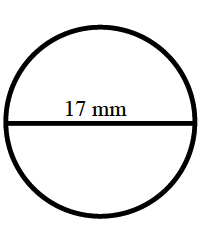### Home > CC3MN > Chapter 4 > Lesson 4.1.5 > Problem4-46

4-46.

Find the area and circumference of a circle that has a diameter of $17$ mm. Write your answers in terms of $\pi$ and as a decimal approximation.

Use the formulas for the area and circumference of a circle:

Area: $\pi·r^2$

Circumference: $\pi·2r$ or $\pi·d$$\text{A }=\pi(8.5)2=72.25\pi\approx226.98\text{ mm}^2$

$\text{C }=2\pi(8.5)\approx53.4\text{ mm}$